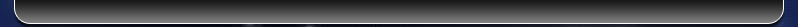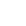# FAQ's for Sensitizing Dyes

### What is absorptivity?

Absorptivity is a measure of the amount of light absorbed by a solution.  Usually defined by Beer’s Law, absorptivity is proportional to the concentration of the absorbing solute.

molar absorptivity is absorptivity defined in terms of concentrations expressed in moles per liter. Symbol e.

e=A/cl    c=M(moles/liter)

e has the units of L mol-1 cm-1

specific absorptivity is absorptivity defined in terms of concentrations expressed in grams per liter. Symbol a.

a =A/cl    c=grams/liter